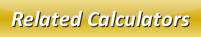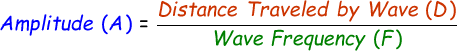# Amplitude Calculator

Amplitude is the utmost height observed in the wave. The amplitude is measured in decibels and is denoted by A.

Formula to calculate amplitude of a wave is given by:where,
A = Amplitude of the wave [decibels]
D = Distance traveled by the wave [meters]
F = Wave frequency [hertz]

Enter the distance traveled by the wave and wave frequency in our below online amplitude calculator and click calculate button to find the answer.

 Distance Traveled by Wave (D): [meters] Wave Frequency (F): [hertz] Amplitude of the Wave (A): [decibels]

Related Calculator: Wavelength to Frequency Converter

Latest Calculator Release

Average Acceleration Calculator

Average acceleration is the object's change in speed for a specific given time period. ...

Free Fall Calculator

When an object falls into the ground due to planet's own gravitational force is known a...

Torque Calculator

Torque is nothing but a rotational force. In other words, the amount of force applied t...

Average Force Calculator

Average force can be explained as the amount of force exerted by the body moving at giv...

Angular Displacement Calculator

Angular displacement is the angle at which an object moves on a circular path. It is de...